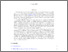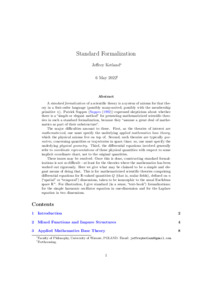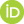# Standard Formalization

Jeffrey Ketland, Jeffrey Ketland (2022) Standard Formalization. [Preprint]Preview
Text
SF.pdf

## Abstract

A \emph{standard formalization} of a scientific theory is a system of axioms for that theory in a first-order language (possibly many-sorted; possibly with the membership primitive $\in$). Patrick Suppes (\cite{sup92}) expressed skepticism about whether there is a simple or elegant method'' for presenting mathematicized scientific theories in such a standard formalization, because they assume a great deal of mathematics as part of their substructure''.

The major difficulties amount to these. First, as the theories of interest are \emph{mathematicized}, one must specify the underlying \emph{applied mathematics base theory}, which the physical axioms live on top of. Second, such theories are typically \emph{geometric}, concerning quantities or trajectories in space/time: so, one must specify the underlying \emph{physical geometry}. Third, the differential equations involved generally refer to \emph{coordinate representations} of these physical quantities with respect to some implicit coordinate chart, not to the original quantities.

These issues may be resolved. Once this is done, constructing standard formalizations is not so difficult---at least for the theories where the mathematics has been worked out rigorously. Here we give what may be claimed to be a simple and elegant means of doing that. This is for mathematicized scientific theories comprising differential equations for $\R$-valued quantities $Q$ (that is, scalar fields), defined on $n$ (spatial'' or temporal'') dimensions, taken to be isomorphic to the usual Euclidean space $\R^n$. For illustration, I give standard (in a sense, text-book'') formalizations: for the simple harmonic oscillator equation in one-dimension and for the Laplace equation in two dimensions.

 Export/Citation: EndNote | BibTeX | Dublin Core | ASCII/Text Citation (Chicago) | HTML Citation | OpenURL
 Social Networking:

Item Type: Preprint
Creators:
CreatorsEmailORCIDJeffrey Ketland, Jeffrey Ketlandjeffreyketland@gmail.com0000-0002-5128-4387
Keywords: Formalization of scientific theories
Subjects: General Issues > Structure of Theories
Depositing User: Dr Jeffrey Ketland
Date Deposited: 09 May 2022 04:07
Item ID: 20559
Subjects: General Issues > Structure of Theories
Date: 6 May 2022
URI: http://philsci-archive.pitt.edu/id/eprint/20559

### Monthly Views for the past 3 yearsView Item